# NumPy Array manipulation: roll() function

## numpy.roll() function

The roll() function is used to roll array elements along a given axis.
Elements that roll beyond the last position are re-introduced at the first.

Syntax:

`numpy.roll(a, shift, axis=None)`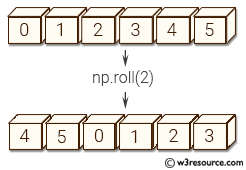Version: 1.15.0

Parameter:

Name Description Required /
Optional
a Input array. Required
shift The number of places by which elements are shifted. If a tuple, then axis must be a tuple of the same size, and each of the given axes is shifted by the corresponding number. If an int while axis is a tuple of ints, then the same value is used for all given axes. Required
axis Axis or axes along which elements are shifted. By default, the array is flattened before shifting, after which the original shape is restored. Optional

Return value:

res : ndarray - Output array, with the same shape as a.

Example-1: numpy.roll() function

``````>>> import numpy as np
>>> a = np.arange(8)
>>> np.roll(a, 3)
array([5, 6, 7, 0, 1, 2, 3, 4])
``````

Pictorial Presentation: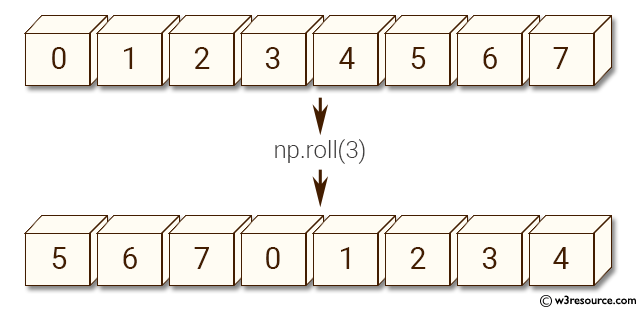Example-2: numpy.roll() function

``````>>> import numpy as np
>>> a = np.arange(8)
>>> b = np.reshape(a, (2, 4))
>>> b
array([[0, 1, 2, 3],
[4, 5, 6, 7]])
>>> np.roll(b, 1)
array([[7, 0, 1, 2],
[3, 4, 5, 6]])
``````

Pictorial Presentation: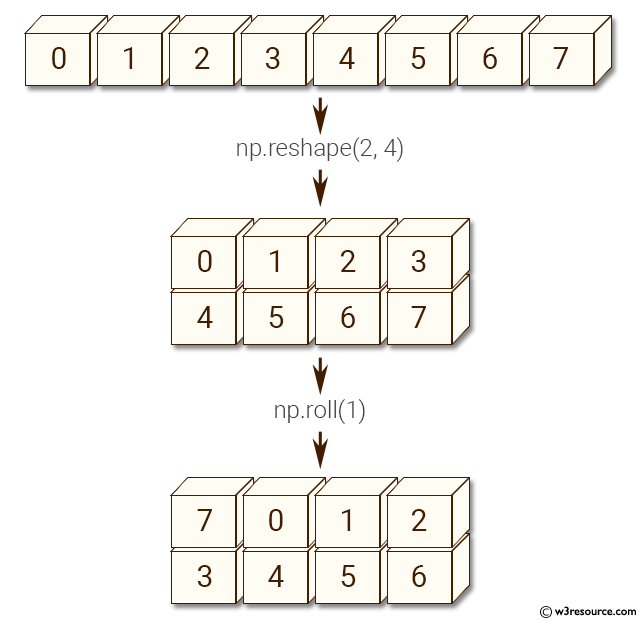Example-3: numpy.roll() function

``````>>> import numpy as np
>>> a = np.arange(8)
>>> b = np.reshape(a, (2, 4))
>>> np.roll(b, 1, axis=0)
array([[4, 5, 6, 7],
[0, 1, 2, 3]])
``````

Pictorial Presentation: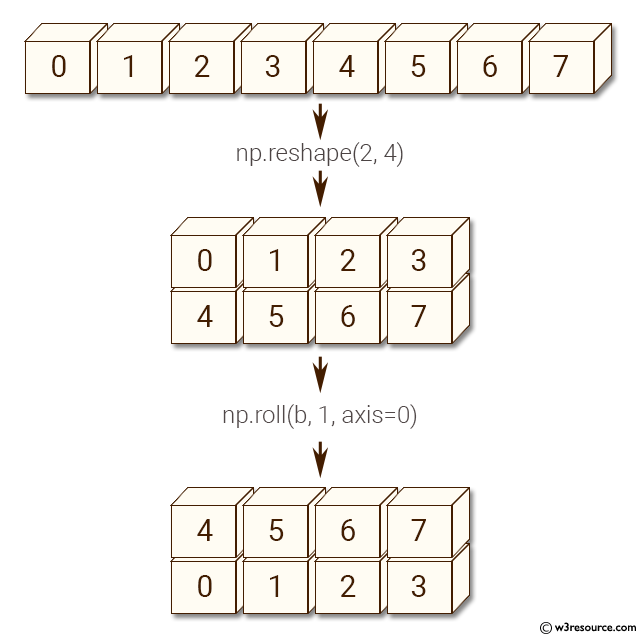Example-4: numpy.roll() function

``````>>> import numpy as np
>>> a = np.arange(8)
>>> b = np.reshape(a, (2, 4))
>>> np.roll(b, 1, axis=1)
array([[3, 0, 1, 2],
[7, 4, 5, 6]])
``````

Pictorial Presentation: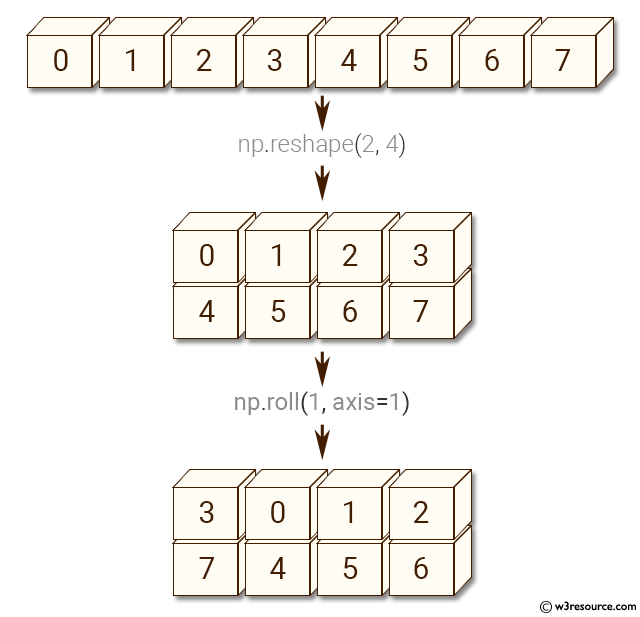Python - NumPy Code Editor:

Previous: flipud()
Next: rot90()

﻿